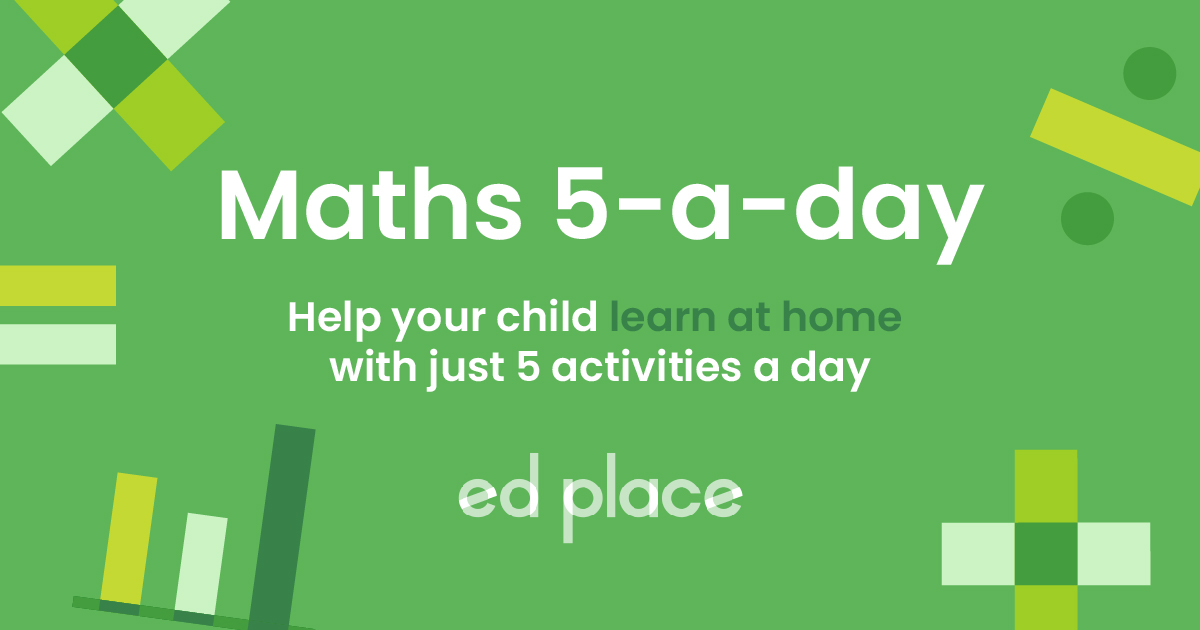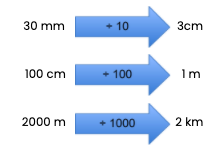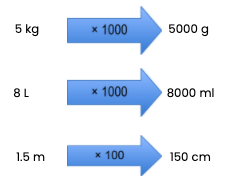# EdPlace's Year 3 and 4 home learning maths lesson: Converting between units of measure (metric)

Looking for short lessons to keep your child engaged and learning? Our experienced team of teachers have created English, maths and science lessons for the home, so your child can learn no matter where they are.  And, as all activities are self-marked, you really can encourage your child to be an independent learner.

Get them started on the lesson below and then jump into our teacher-created activities to practice what they've learnt. We've recommended five to ensure they feel secure in their knowledge - 5-a-day helps keeps the learning loss at bay (or so we think!).

Are they keen to start practising straight away? Head to the bottom of the page to find the activities.

Now...onto the lesson!

Key Stage 2 Statutory Requirements for Maths
Year 3 and 4  students should be able to convert between different units of measure (e.g kilometre to metre).

When was the last time you had to convert millimetres to centimetres or kilometres to metres? As adults, we can often do this very easily mentally but it’s a common area of confusion for children who struggle with mental maths methods and understanding of place value.

Why not check out our step by step approach?

We're confident that if you follow this step-by-step approach together, your child will be able to:

1) Understand how to convert between metric units of measure including millimetres, centimetres, kilometres and metres.

2) Apply this understanding to convert smaller units into larger units and vice versa

3) Explain how to solve some simple word problems involving measures, if they've really cracked it!

## Step 1 - Key Terminology

Before we jump into converting measurements it’s important to check that your child understands what the key terminology means.

We use abbreviations when writing about measurements:

We can measure length in kilometres (km), metres (m), centimetres (cm) or millimetres (mm).

Mass (or weight) is measured in grams (g) or kilograms (kg).

Capacity is measured in litres (L) or millilitres (ml)

## Step 2 - Unit of Measure

When we measure objects, liquids or distances, it is important to use a sensible unit of measure

For example, when measuring car journey lengths, km are far more sensible than m, cm or mm! However, we would use metres when measuring the length of the garden and cm or mm for measuring smaller items such as pencils or small toys.

In year 3, your child will have learned some very simple equivalent measurements, such as 10mm = 1 cm and 100cm = 1 m. They will also have measured lengths in cm and mm e.g a pencil could be 16 cm and 4 mm.

To be able to convert between units, children must also have a really sound understanding of place value and what happens when we divide and multiply by 10, 100 and 1000.

## Step 3 - Converting Between Measurements

When converting between two types of measurements, it is useful to know some basic facts.

1 cm = 10 mm

1 m = 100 cm

1 km = 1000 m

1 L = 1000 g

1 kg = 1000 g

Do you notice that these facts involve multiples of 10, 100 and 1000? This is really important. When converting between metric measures, we always multiply or divide by 10, 100 or 1000.

When converting from a smaller unit to a larger unit - we always divide!

E.g.When converting from a larger unit to a smaller unit - we always multiply!

E.g.## Step 4 - Putting it into Practice

Why not apply the above to the following measurement questions together?

1. How many metres are there in 3km?

2. How many cm in 55mm?

3. James has 3 bags of flour each with a mass of 2kg. How many grams of flour does he have in total?

4. Chloe has a 2 litre jug of orange and drinks 1500ml. How much is left in the jug?

## Step 5 - Give it a go...

Why not test your child's understanding and see if they can tackle these activities?

All activities are created by teachers and automatically marked. Plus, with an EdPlace subscription, we can automatically progress your child at a level that's right for them. Sending you progress reports along the way so you can track and measure progress, together - brilliant!

1. 3000 metres.

2. 5.5 or 5 ½ cm.

3. 6000 grams.

4. 500 ml or ½ litre.

Keep going! Looking for more activities, different subjects or year groups?

Click the button below to view the EdPlace English, maths, science and 11+ activity library